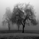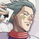12872 views
Period= Length of the slow HA
Fastend and Slowend = just calculations for the Kama function no need to change those.
Signal= Shows/Hides the triangles
```study("Slow Heiken Ashi",overlay=true,precision=0)
//by Glaz.
//KAMA function
p=input(6,title='Period')
fastend=input(0.666,step=0.001)
slowend=input(0.0645,step=0.0001)
kama(close,amaLength)=>
diff=abs(close-close)
signal=abs(close-close[amaLength])
noise=sum(diff, amaLength)
efratio=noise!=0 ? signal/noise : 1
smooth=pow(efratio*(fastend-slowend)+slowend,2)
kama=nz(kama, close)+smooth*(close-nz(kama, close))
kama

//Slow Heiken Ashi
hakamaper=1/2
Signal=input(true)
Om=sma(open,p)
Hm=sma(high,p)
Lm=sma(low,p)
Cm=sma(close,p)
vClose=(Om+Hm+Lm+Cm)/4
vOpen= kama(vClose,hakamaper)
vHigh= max(Hm,max(vClose, vOpen))
vLow=  min(Lm,min(vClose, vOpen))

// Plots
vcolor= vOpen>vClose ?red:green
plotcandle(vOpen,vHigh,vLow,vClose,color=vcolor)

//signals
plotchar(Signal?(cross(vOpen,vClose) and vOpen<vClose?vHigh:na):na,char='▼',color=white,transp=0,location=location.absolute)
plotchar(Signal?(cross(vOpen,vClose) and vOpen>vClose?vLow:na):na,char='▲',color=white,transp=0,location=location.absolute)I'm getting this when trying to apply...

Invalid value of the `length’ argument (0) `sum’ in the function. It should be >0

How do i remedy?Mohi1006
@Mohi1006,
Hello.
I have tried doing this but it's still saying `length’ argument (0) `sum’ in the function. It should be >0'
Do you have to activate this change once you have altered the code?
Many thanks

P.S. I just discovered you have to reapply the indicator to the chart then it works fineHi Glaz.
Love this script. Nice job.
Just a litter contribution . Add this couples of lines in order to have the signal Alert.

short= cross(vOpen,vClose) and vOpen<vClose?vHigh:na
long= cross(vOpen,vClose) and vOpen>vClose?vLow:na